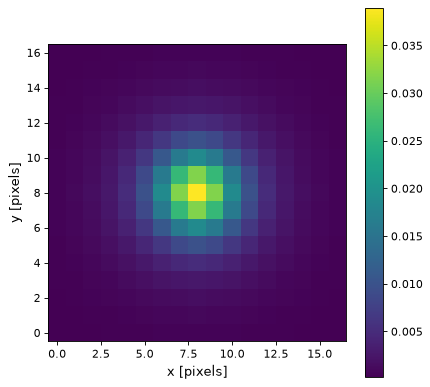# Moffat2DKernel¶

class astropy.convolution.Moffat2DKernel(gamma, alpha, **kwargs)[source]

2D Moffat kernel.

This kernel is a typical model for a seeing limited PSF.

Parameters
gammafloat

Core width of the Moffat model.

alphafloat

Power index of the Moffat model.

x_sizeodd int, optional

Size in x direction of the kernel array. Default = 8 * radius.

y_sizeodd int, optional

Size in y direction of the kernel array. Default = 8 * radius.

modestr, optional
One of the following discretization modes:
• ‘center’ (default)

Discretize model by taking the value at the center of the bin.

• ‘linear_interp’

Discretize model by performing a bilinear interpolation between the values at the corners of the bin.

• ‘oversample’

Discretize model by taking the average on an oversampled grid.

• ‘integrate’

Discretize model by integrating the model over the bin.

factornumber, optional

Factor of oversampling. Default factor = 10.

Examples

Kernel response:

import matplotlib.pyplot as plt
from astropy.convolution import Moffat2DKernel
moffat_2D_kernel = Moffat2DKernel(3, 2)
plt.imshow(moffat_2D_kernel, interpolation='none', origin='lower')
plt.xlabel('x [pixels]')
plt.ylabel('y [pixels]')
plt.colorbar()
plt.show()


(png, svg, pdf)